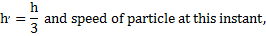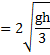# A particle is released from a height h, At a certain height; its KE is two times its potential energy. Height and speed of the particle at that instant are a)b)c)d)## Question ID - 50977 :- A particle is released from a height h, At a certain height; its KE is two times its potential energy. Height and speed of the particle at that instant are a)b)c)d)3537

(b)

Total mechanical energy= mgh

As,andHeight from the ground at this instant,Next Question :
 A particle is placed at the origin and a forceis acting on it (whereis positive constant). If, the graph ofversuswill be (whereis the potential energy function) a)b)c)d)